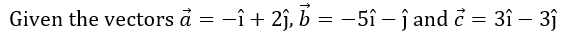# Adding and subtracting vectors in component form - Vectors

### Adding and subtracting vectors in component form

In this section, we will learn how to find the sum, as well as the difference between vectors algebraically and graphically. We will do so with two methods – the "Tip To Tail" method, and the "parallelogram method.

#### Lessons

#####